Related Articles

# Setting Different error bar colors in bar plot in Matplotlib

• Last Updated : 03 Jan, 2021

Python provides us with a variety of libraries where Matplotlib is one of them. It is used for data visualization purposes. In this article, we will be setting different error bar colors in the bar plot in Matplotlib.

## Error bars in Matplotlib

Various plots of matplotlib such as bar charts, line plots can use error bars. Error bars are used for showing the precision in measurements or calculated values. Without Error Bars, the plot created using matplotlib from a set of values looks to be of high precision or high confidence.

Attention geek! Strengthen your foundations with the Python Programming Foundation Course and learn the basics.

To begin with, your interview preparations Enhance your Data Structures concepts with the Python DS Course. And to begin with your Machine Learning Journey, join the Machine Learning - Basic Level Course

Syntax: matplotlib.pyplot.errorbar(x, y, yerr=None, xerr=None, fmt=”, ecolor=None, elinewidth=None, capsize=None, barsabove=False, lolims=False, uplims=False, xlolims=False, xuplims=False, errorevery=1, capthick=None, \*, data=None, \*\*kwargs)

Parameters: This method accept the following parameters that are described below:

• x, y: These parameters are the horizontal and vertical coordinates of the data points.
• ecolor: This parameter is an optional parameter. And it is the color of the errorbar lines with default value NONE.
• elinewidth: This parameter is also an optional parameter. And it is the linewidth of the errorbar lines with default value NONE.
• capsize: This parameter is also an optional parameter. And it is the length of the error bar caps in points with default value NONE.
• barsabove: This parameter is also an optional parameter. It contains boolean value True for plotting errorsbars above the plot symbols.Its default value is False.

## How to set different error bar colors in bar plot in Matplotlib

Example 1:

Step 1: Create a bar plot at first.

## Python3

 `# import matplotlib package``import` `matplotlib.pyplot as plt`` ` `# Store set of values in x ``# and height for plotting ``# the graph``x ``=` `range``(``4``)``height ``=` `[ ``3``, ``6``, ``5``, ``4``]`` ` `# using tuple unpacking``# to grab fig and axes``fig, ax ``=` `plt.subplots()`` ` `# Creating the bar plot ``# with opacity=0.1``ax.bar(x, height, alpha ``=` `0.1``)`

Output: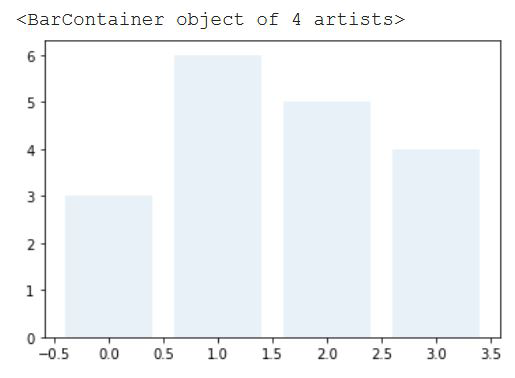Step 2: Add Error Bar to each of the points:

## Python3

 `# import matplotlib package``import` `matplotlib.pyplot as plt`` ` `# Store set of values in x ``# and height for plotting ``# the graph``x``=` `range``(``4``)``height``=``[ ``3``, ``6``, ``5``, ``4``]`` ` `# using tuple unpacking``# to grab fig and axes``fig, ax ``=` `plt.subplots()`` ` `# Creating the bar plot ``# with opacity=0.1``ax.bar(x, height, alpha ``=` `0.1``)`` ` `# Zip function acts as an``# iterator for tuples so that``# we are iterating through ``# each set of values in a loop`` ` `for` `pos, y, err ``in` `zip``(x, height, error):``    ``ax.errorbar(pos, y, err, lw ``=` `2``,``                ``capsize ``=` `4``, capthick ``=` `4``, ``                ``color ``=` `"green"``)``     ` `# Showing the plotted error bar``# plot with same color which is``# green``plt.show()`

Output: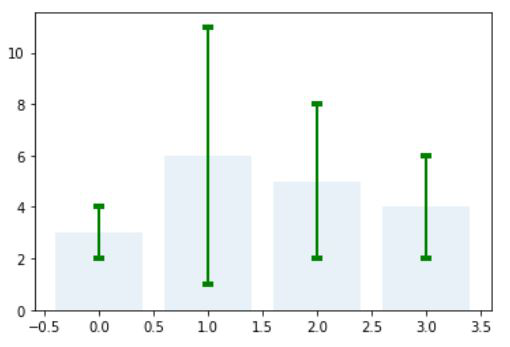Step 3: Setting different error bar colors in the bar plot(Example 1):

## Python3

 `# importing matplotlib``import` `matplotlib.pyplot as plt`` ` `# Storing set of values in``# x, height, error and colors for ploting the graph``x``=` `range``(``4``)``height``=``[ ``3``, ``6``, ``5``, ``4``]``error``=``[ ``1``, ``5``, ``3``, ``2``]``colors ``=` `[``'red'``, ``'green'``, ``'blue'``, ``'black'``]`` ` `# using tuple unpacking``# to grab fig and axes``fig, ax ``=` `plt.subplots()`` ` `# ploting the bar plot``ax.bar( x, height, alpha ``=` `0.1``)`` ` `# Zip function acts as an``# iterator for tuples so that``# we are iterating through ``# each set of values in a loop``for` `pos, y, err, colors ``in` `zip``(x, height, ``                               ``error, colors):``   ` `    ``ax.errorbar(pos, y, err, lw ``=` `2``,``                ``capsize ``=` `4``, capthick ``=` `4``, ``                ``color ``=` `colors)``     ` `# Showing the plotted error bar``# plot with different color ``plt.show()`

Output: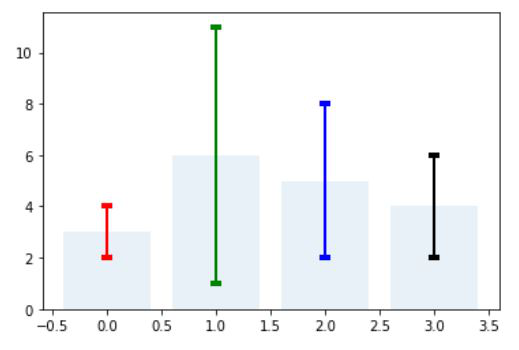Example 2: Setting different error bar colors in the bar plot:

## Python3

 `# importing matplotlib package``import` `matplotlib.pyplot as plt`` ` `# importing the numpy package``import` `numpy as np`` ` `# Storing set of values in``# names, x, height, ``# error and colors for ploting the graph``names``=` `[``'Bijon'``, ``'Sujit'``, ``'Sayan'``, ``'Saikat'``]``x``=``np.arange(``4``)``marks``=``[ ``60``, ``90``, ``55``, ``46``]``error``=``[ ``11``, ``15``, ``5``, ``9``]``colors ``=` `[``'red'``, ``'green'``, ``'blue'``, ``'black'``]`` ` `# using tuple unpacking``# to grab fig and axes``fig, ax ``=` `plt.subplots()`` ` `# ploting the bar plot``ax.bar(x, marks, alpha ``=` `0.5``,``       ``color ``=` `colors)`` ` `# Zip function acts as an``# iterator for tuples so that``# we are iterating through ``# each set of values in a loop``for` `pos, y, err, colors ``in` `zip``(x, marks,``                               ``error, colors):``   ` `    ``ax.errorbar(pos, y, err, lw ``=` `2``,``                ``capsize ``=` `4``, capthick ``=` `4``,``                ``color ``=` `colors)``     ` `# Showing the plotted error bar``# plot with different color ``ax.set_ylabel(``'Marks of the Students'``)`` ` `# Using x_ticks and x_labels``# to set the name of the``# students at each point``ax.set_xticks(x)``ax.set_xticklabels(names)``ax.set_xlabel(``'Name of the students'``)`` ` `# Showing the plot``plt.show()`

Output: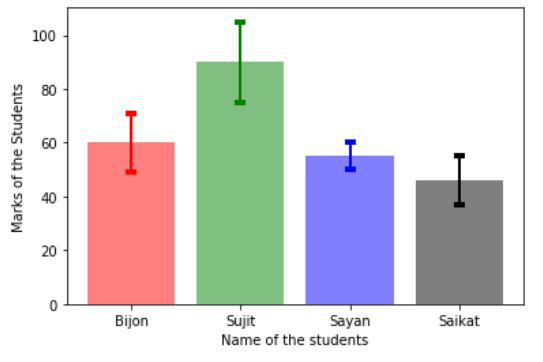Example 3: Setting different error bar colors in the bar plot.

## Python3

 `# importing matplotlib``import` `matplotlib.pyplot as plt`` ` `# importing the numpy package``import` `numpy as np`` ` `# Storing set of values in``# names, x, height, error, ``# error1 and colors for ploting the graph``names``=` `[``'USA'``, ``'India'``, ``'England'``, ``'China'``]``x``=``np.arange(``4``)``economy``=``[``21.43``, ``2.87``, ``2.83``, ``14.34``]``error``=``[``1.4``, ``1.5``, ``0.5``, ``1.9``]``error1``=``[``0.5``, ``0.2``, ``0.6``, ``1``]``colors ``=` `[``'red'``, ``'grey'``, ``'blue'``, ``'magenta'``]`` ` `# using tuple unpacking``# to grab fig and axes``fig, ax ``=` `plt.subplots()`` ` `# ploting the bar plot``ax.bar(x, economy, alpha ``=` `0.5``,``       ``color ``=` `colors)`` ` `# Zip function acts as an``# iterator for tuples so that``# we are iterating through ``# each set of values in a loop``for` `pos, y, err,err1, colors ``in` `zip``(x, economy,``                                    ``error, error1, ``                                    ``colors):``   ` `    ``ax.errorbar(pos, y, err, err1, fmt ``=` `'o'``,``                ``lw ``=` `2``, capsize ``=` `4``, capthick ``=` `4``,``                ``color ``=` `colors)``     ` `# Showing the plotted error bar``# plot with different color ``ax.set_ylabel(``'Economy(in trillions)'``)`` ` `# Using x_ticks and x_labels``# to set the name of the``# countries at each point``ax.set_xticks(x)``ax.set_xticklabels(names)``ax.set_xlabel(``'Name of the countries'``)`` ` `# Showing the plot``plt.show()`

Output: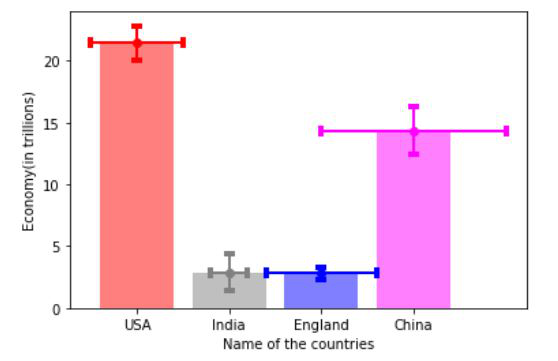My Personal Notes arrow_drop_up